### Transitive Closure

The transitive closure of a graph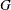is a graph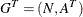such that for all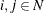there is a link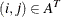if and only if there exists a path fromtoin.

The transitive closure of a graph can help to efficiently answer questions about reachability. Suppose you want to answer the question of whether you can get from nodeto nodein the original graph. Given the transitive closure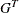of, you can simply check for the existence of link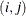to answer the question. This has many applications, including speeding up the processing of structured query languages, which are often used in databases.

In PROC OPTNET, the transitive closure algorithm can be invoked by using the TRANSITIVE_CLOSURE statement. The options for this statement are described in the section TRANSITIVE_CLOSURE Statement.

The results for the transitive closure algorithm are written to the output data set that is specified in the OUT= option in the TRANSITIVE_CLOSURE statement. The links that define the transitive closure are listed in the output data set with variable names from and to.

The algorithm used by PROC OPTNET to compute transitive closure is a sparse version of the Floyd-Warshall algorithm (Cormen, Leiserson, and Rivest 1990). This algorithm runs in time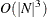and therefore might not scale to very large graphs.

#### Transitive Closure of a Simple Directed Graph

This example illustrates the use of the transitive closure algorithm on the simple directed graphshown in Figure 2.56.

Figure 2.56: A Simple Directed Graph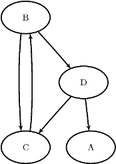The directed graphcan be represented by the links data set LinkSetIn as follows:

data LinkSetIn;
input from $to$ @@;
datalines;
B C  B D  C B  D A  D C
;


The following statements calculate the transitive closure and output the results in the data set TransClosure:

proc optnet
graph_direction = directed
transitive_closure
out          = TransClosure;
run;


The data set TransClosure contains the transitive closure ofand is shown in Figure 2.57.

Figure 2.57: Transitive Closure of a Simple Directed Graph

 Transitive Closure

from to
B C
B D
C B
D A
D C
C C
C D
B B
D B
D D
B A
C A

The transitive closure ofis shown graphically in Figure 2.58.

Figure 2.58: Transitive Closure of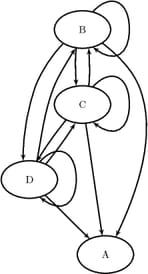For a more detailed example, see Example 2.6.